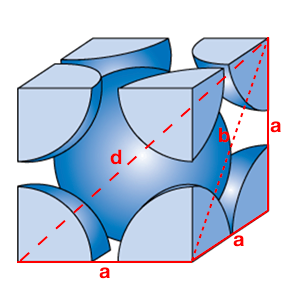# Problem: When spheres of radius r are packed in a body-centered cubic arrangement, they occupy 68 % of the available volume. Use the fraction of occupied volume to calculate the value of a, the length of the edge of the cube in terms of r.

###### FREE Expert Solution

Recall: The body-centered cubic cell contains one atom in the center of the cube and an atom in each of the corners. It looks like this:If r = radius of the sphere, then the diagonal d = 4r, since it spans through 2 spheres. We can express the diagonal b in terms of the length of the cube a, using Pythagorean theorem.

80% (132 ratings)###### Problem Details

When spheres of radius r are packed in a body-centered cubic arrangement, they occupy 68 % of the available volume. Use the fraction of occupied volume to calculate the value of a, the length of the edge of the cube in terms of r.